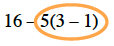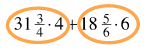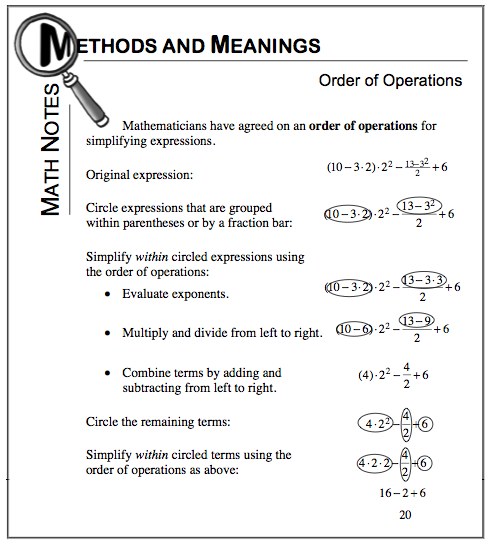### Home > CC1 > Chapter 6 > Lesson 6.2.1 > Problem6-72

6-72.

Simplify each expression. Homework Help ✎

1. $16 - 5 ( 3 - 1 )$

• The image below shows your first step.
Refer to the Math Notes box below if you need more help.

•• $16 − 5(2)$
$16 − 10 = 6$

1. $31 \frac { 3 } { 4 } \cdot 4 + 18 \frac { 5 } { 6 } \cdot 6$

• Complete the circled areas first.

•• $127 + 113 = 240$

1. $3.45 ( 2 ^ { 2 } ) - 8.18 ( 1 )$

• For parts (c) and (d), refer to the Math Notes box and parts (a) or (b).

1. $4 \cdot 5 - 2 ^ { 2 } + 3 ( 5 - 4 )$